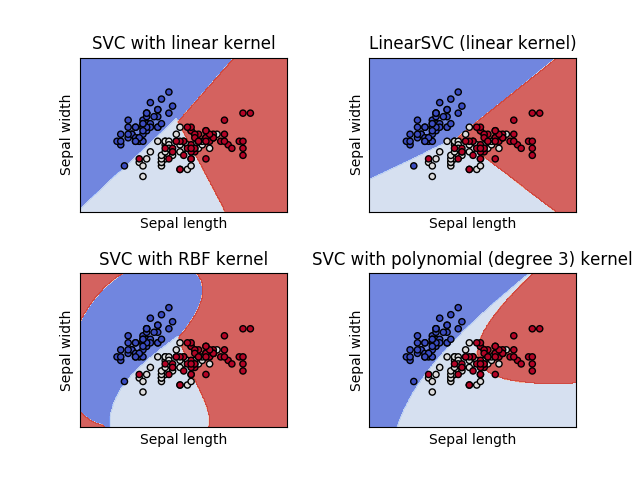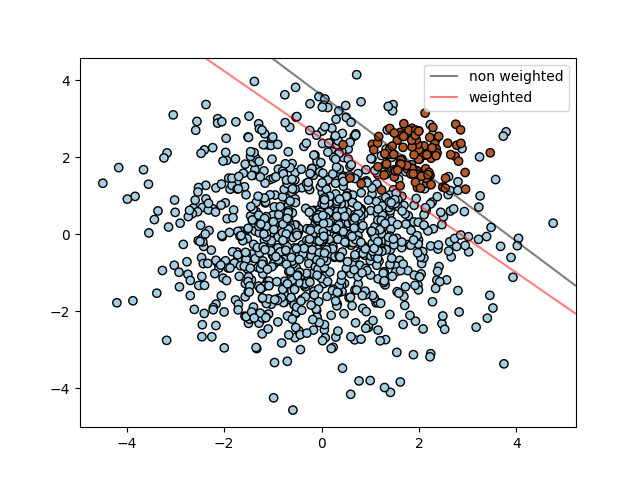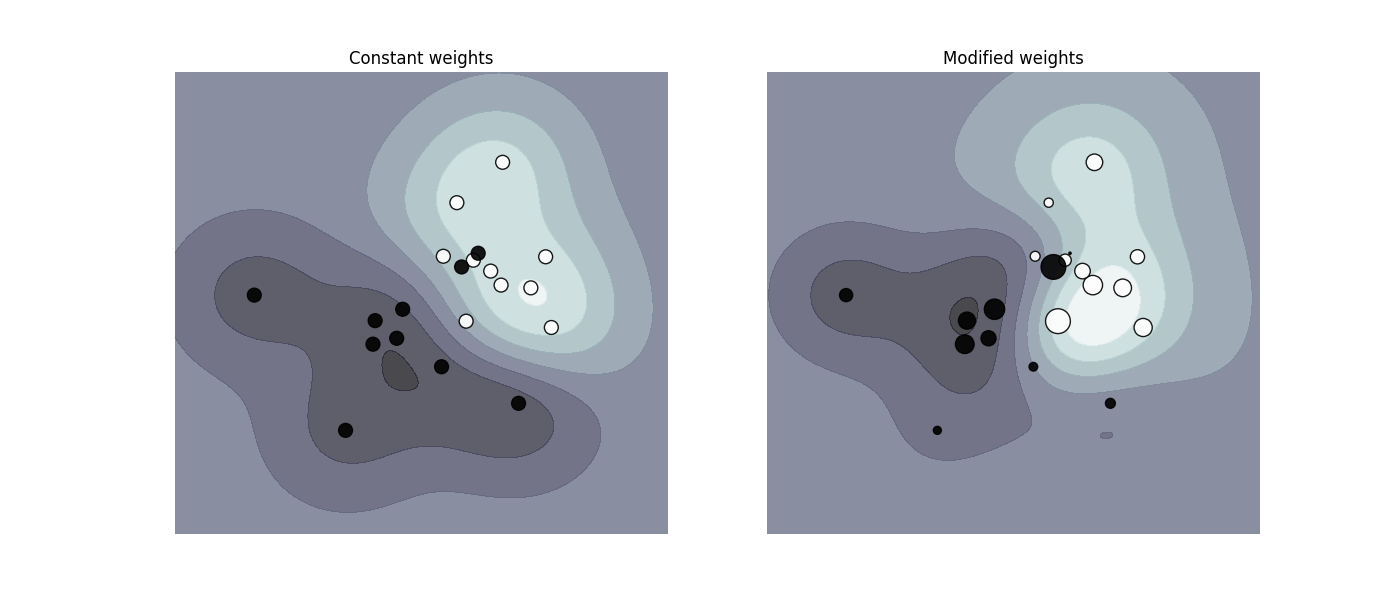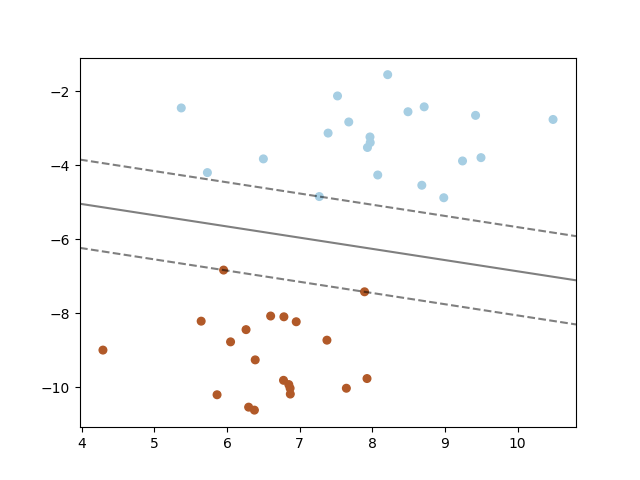# 1.4. 支持向量机

• 在高维空间中非常高效.
• 即使在数据维度比样本数量大的情况下仍然有效.
• 在决策函数（称为支持向量）中使用训练集的子集,因此它也是高效利用内存的.
• 通用性: 不同的核函数 核函数 与特定的决策函数一一对应.常见的 kernel 已经提供,也可以指定定制的内核.

• 如果特征数量比样本数量大得多,在选择核函数 核函数 时要避免过拟合, 而且正则化项是非常重要的.

• 支持向量机不直接提供概率估计,这些都是使用昂贵的五次交叉验算计算的. (详情见 得分和概率).

## 1.4.1. 分类

SVC, NuSVCLinearSVC 能在数据集中实现多元分类.SVCNuSVC 是相似的方法, 但是接受稍许不同的参数设置并且有不同的数学方程(在这部分看 数学公式). 另一方面, LinearSVC 是另一个实现线性核函数的支持向量分类. 记住 LinearSVC 不接受关键词 kernel, 因为它被假设为线性的. 它也缺少一些 SVCNuSVC 的成员(members) 比如 support_ .

>>> from sklearn import svm
>>> X = [[0, 0], [1, 1]]
>>> y = [0, 1]
>>> clf = svm.SVC(gamma='scale')
>>> clf.fit(X, y)
SVC(C=1.0, cache_size=200, class_weight=None, coef0=0.0,
decision_function_shape='ovr', degree=3, gamma='scale', kernel='rbf',
max_iter=-1, probability=False, random_state=None, shrinking=True,
tol=0.001, verbose=False)



>>> clf.predict([[2., 2.]])
array()



SVMs 决策函数取决于训练集的一些子集, 称作支持向量. 这些支持向量的部分特性可以在 support_vectors_, support_n_support 找到:

>>> # 获得支持向量
>>> clf.support_vectors_
array([[ 0.,  0.],
[ 1.,  1.]])
>>> # 获得支持向量的索引
>>> clf.support_
array([0, 1]...)
>>> # 为每一个类别获得支持向量的数量
>>> clf.n_support_
array([1, 1]...)



### 1.4.1.1. 多元分类

SVCNuSVC 为多元分类实现了 “one-against-one” 的方法 (Knerr et al., 1990) 如果 n_class 是类别的数量, 那么 n_class * (n_class - 1) / 2 分类器被重构, 而且每一个从两个类别中训练数据. 为了提供与其他分类器一致的接口, decision_function_shape 选项允许聚合 “one-against-one” 分类器的结果成 (n_samples, n_classes) 的大小到决策函数:

>>> X = [, , , ]
>>> Y = [0, 1, 2, 3]
>>> clf = svm.SVC(gamma='scale', decision_function_shape='ovo')
>>> clf.fit(X, Y)
SVC(C=1.0, cache_size=200, class_weight=None, coef0=0.0,
decision_function_shape='ovo', degree=3, gamma='scale', kernel='rbf',
max_iter=-1, probability=False, random_state=None, shrinking=True,
tol=0.001, verbose=False)
>>> dec = clf.decision_function([])
>>> dec.shape # 4 classes: 4*3/2 = 6
6
>>> clf.decision_function_shape = "ovr"
>>> dec = clf.decision_function([])
>>> dec.shape # 4 classes
4



>>> lin_clf = svm.LinearSVC()
>>> lin_clf.fit(X, Y)
LinearSVC(C=1.0, class_weight=None, dual=True, fit_intercept=True,
intercept_scaling=1, loss='squared_hinge', max_iter=1000,
multi_class='ovr', penalty='l2', random_state=None, tol=0.0001,
verbose=0)
>>> dec = lin_clf.decision_function([])
>>> dec.shape
4



dual_coef_ 的形状是 [n_class-1, n_SV], 这个结构有些难以理解. 对应于支持向量的列与 n_class * (n_class - 1) / 2 “one-vs-one” 分类器相关. 每一个支持向量用于 n_class - 1 分类器中.对于这些分类器,每一行的 n_class - 1 条目对应于对偶系数(dual coefficients).第0类的SVs系数第1类的SVs系数第2类的SVs系数(空单元格表示与上方单元格合并)

### 1.4.1.2. 得分和概率

SVC 方法的 decision_function 给每一个样例每一个类别分值(scores)(或者在一个二元类中每一个样例一个分值). 当构造器(constructor)选项 probability 设置为 True 的时候, 类成员可能性评估开启.(来自 predict_probapredict_log_proba 方法) 在二元分类中,概率使用 Platt scaling 进行标准化: 在 SVM 分数上的逻辑回归,在训练集上用额外的交叉验证来拟合.在多类情况下,这可以扩展为 per Wu et al.(2004)

### 1.4.1.3. 非均衡问题

SVC (而不是 NuSVC) 在 fit 方法中生成了一个关键词 class_weight. 它是形如 {class_label : value} 的字典, value 是浮点数大于 0 的值, 把类 class_label 的参数 C 设置为 C * value.SVC, NuSVC, SVR, NuSVROneClassSVMfit 方法中通过关键词 sample_weight 为单一样例实现权重weights.与 class_weight 相似, 这些把第i个样例的参数 C 换成 C * sample_weight[i].## 1.4.2. 回归

>>> from sklearn import svm
>>> X = [[0, 0], [2, 2]]
>>> y = [0.5, 2.5]
>>> clf = svm.SVR()
>>> clf.fit(X, y)
SVR(C=1.0, cache_size=200, coef0=0.0, degree=3, epsilon=0.1, gamma='auto_deprecated',
kernel='rbf', max_iter=-1, shrinking=True, tol=0.001, verbose=False)
>>> clf.predict([[1, 1]])
array([ 1.5])



## 1.4.3. 密度估计, 异常（novelty）检测

OneClassSVM实现了一个用于离群点检测的单类SVM。

## 1.4.5. 使用诀窍

• 避免数据复制: 对于 SVCSVRNuSVCNuSVR， 如果数据是通过某些方法而不是用行优先存储（C-order）的双精度，那它会在调用底层的 C 命令前先被复制。 您可以通过检查它的 flags 属性，来确定给定的 numpy 数组是不是行优先存储（C-order）的。

对于 LinearSVC (和 LogisticRegression) 的任何输入，都会以 numpy 数组形式，被复制和转换为 用 liblinear 内部稀疏数据去表达（双精度浮点型 float 和非零部分的 int32 索引）。 如果您想要一个适合大规模的线性分类器，又不打算复制一个密集的行优先存储（C-order）双精度 numpy 数组作为输入， 那我们建议您去使用 SGDClassifier 类作为替代。目标函数可以配置为和 LinearSVC 模型差不多相同的。

• 内核的缓存大小: 在大规模问题上，对于 SVC, SVR, nuSVCNuSVR, 内核缓存的大小会特别影响到运行时间。如果您有足够可用的 RAM，不妨把它的 缓存大小 设得比默认的 200(MB) 要高，例如为 500(MB) 或者 1000(MB)。

• 惩罚系数C的设置:在合理的情况下， C 的默认选择为 1 。如果您有很多混杂的观察数据， 您应该要去调小它。 C 越小，就能更好地去正规化估计。

当C值较大时，LinearSVCLinearSVR对C值较不敏感，即当C值大于特定阈值后，模型效果将会停止提升。同时，较大的C值将会导致较长的训练时间，Fan et al.(2008)的论文显示，训练时间的差距有时会达到10倍。

• 支持向量机算法本身不能够很好地支持非标准化的数据，所以 我们强烈建议您将数据标准化。 举个示例，对于输入向量 X， 规整它的每个数值范围为 [0, 1] 或 [-1, +1] ，或者标准化它的为均值为0方差为1的数据分布。请注意， 相同的缩放标准必须要应用到所有的测试向量，从而获得有意义的结果。 请参考章节 预处理数据 ，那里会提供到更多关于缩放和规整。

• NuSVC/OneClassSVM/NuSVR 内的参数 nu ， 近似是训练误差和支持向量的比值。
• SVC, ，如果分类器的数据不均衡（例如，很多正例很少负例），设置 class_weight='balanced' 与/或尝试不同的惩罚系数 C
• 底层实现的随机性:SVCNuSVC的底层实现仅使用随机数生成器来打乱数据顺序进行概率估计(当probability被设置为True时)。这种随机性可以用random_state参数来控制。如果将probability设为False，这些估计器就不是随机的，random_state对结果没有影响。底层的OneClassSVM实现类似于SVCNuSVC的实现。由于OneClassSVM没有提供概率估计，所以它不是随机的。 底层的LinearSVC实现使用随机数生成器来选择特征，当用双坐标下降(当dual被设置为True)。因此，对于相同的输入数据，结果略有不同并不罕见。如果发生这种情况，尝试使用较小的tol参数。这种随机性也可以通过random_state参数来控制。当dual设置为False时，LinearSVC的底层实现不是随机的，random_state对结果没有影响。

• 使用由 LinearSVC(loss='l2', penalty='l1', dual=False) 提供的 L1 惩罚去产生稀疏解，也就是说，特征权重的子集不同于零，这样做有助于决策函数。 随着增加 C 会产生一个更复杂的模型（有更多的特征被选择）。可以使用 l1_min_c 去计算 C 的数值，去产生一个”null” 模型（所有的权重等于零）。

## 1.4.6. 核函数

• 线性:.
• 多项式:.是关键词 degree,指定 coef0
• rbf:.是关键词 gamma, 必须大于 0。
• sigmoid (), 其中指定 coef0

>>> linear_svc = svm.SVC(kernel='linear')
>>> linear_svc.kernel
'linear'
>>> rbf_svc = svm.SVC(kernel='rbf')
>>> rbf_svc.kernel
'rbf'



### 1.4.6.1. 自定义核

• 空间 support_vectors_ 现在是空的, 只有支持向量的索引被存储在 support_
• fit() 方法的第一个参数的引用（不是副本）将被存储，并作为将来的引用。 如果在 fit()predict() 之间有数组发生改变，您将会碰到意料外的结果。

#### 1.4.6.1.1. 使用 python 函数作为内核

>>> import numpy as np
>>> from sklearn import svm
>>> def my_kernel(X, Y):
...     return np.dot(X, Y.T)
...
>>> clf = svm.SVC(kernel=my_kernel)



#### 1.4.6.1.2. 使用 Gram 矩阵

>>> import numpy as np
>>> from sklearn import svm
>>> X = np.array([[0, 0], [1, 1]])
>>> y = [0, 1]
>>> clf = svm.SVC(kernel='precomputed')
>>> # 线性内核计算
>>> gram = np.dot(X, X.T)
>>> clf.fit(gram, y)
SVC(C=1.0, cache_size=200, class_weight=None, coef0=0.0,
decision_function_shape='ovr', degree=3, gamma='auto_deprecated',
kernel='precomputed', max_iter=-1, probability=False,
random_state=None, shrinking=True, tol=0.001, verbose=False)
>>> # 预测训练样本
>>> clf.predict(gram)
array([0, 1])



## 1.4.7. 数学公式### 1.4.7.1. SVC### 1.4.7.3. SVR